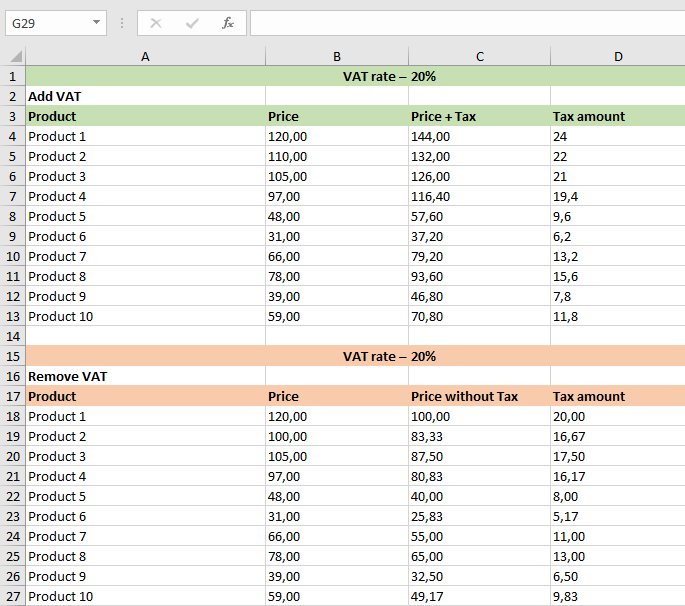# South African VAT Calculator

Updated on May 13, 2022

VAT may be added or removed in a matter of seconds with our VAT calculator. A simple click of the button is all that is required.

## What is VAT and how much is it in South Africa?

European Union Member States are required to collect VAT (known in certain countries as a GST), which is a tax on the sale of goods and services inside the EU. The usual VAT rate is now 15%. There are zero percent VAT reductions available.

Businesses that are registered for VAT can obtain information on how to register for and pay and reclaim VAT at sars.gov.za.

## How to work out VAT

Only 3 easy steps to calculate VAT onlinePlease double-check the “VAT rate” (which is now set at 15%)Specify whether the pricing is net or gross. The full list of VAT rates can be seen here.## How to calculate VAT in math or Excel

You can use these simple procedures to calculate VAT in either direction.Just divide the price by 100 and multiply by (100 + VAT rate) if you wish to add VAT to the price. Including VAT – Gross price, that’s everything you need to know about the pricing.### Removing VAT formula

The price must be divided by (100 + VAT rate) and then multiplied by 100 if you want to subtract VAT from the total price It is now clear to you how much it will cost you excluding VAT – the net price.

Net price.### VAT calculation in Excel

If you need to calculate VAT for several products at once, it is easier to use Excel. I created a template – download it, it’s free.

Categories VAT